### Nonlinear Growth Curves with Gaussian Data

As an introductory example, consider the orange tree data of Draper and Smith (1981). These data consist of seven measurements of the trunk circumference (in millimeters) on each of five orange trees. You can input these data into a SAS data set as follows:

data tree;
input tree day y;
datalines;
1  118   30
1  484   58
1  664   87

... more lines ...

5 1582  177
;


Lindstrom and Bates (1990) and Pinheiro and Bates (1995) propose the following logistic nonlinear mixed model for these data: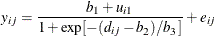Here,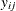represents the jth measurement on the ith tree (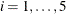;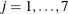),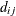is the corresponding day,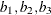are the fixed-effects parameters,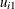are the random-effect parameters assumed to be iid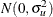, and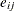are the residual errors assumed to be iid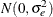and independent of the. This model has a logistic form, and the random-effect parametersenter the model linearly.

The statements to fit this nonlinear mixed model are as follows:

proc nlmixed data=tree;
parms b1=190 b2=700 b3=350 s2u=1000 s2e=60;
num = b1+u1;
ex  = exp(-(day-b2)/b3);
den = 1 + ex;
model y ~ normal(num/den,s2e);
random u1 ~ normal(0,s2u) subject=tree;
run;


The PROC NLMIXED statement invokes the procedure and inputs the tree data set. The PARMS statement identifies the unknown parameters and their starting values. Here there are three fixed-effects parameters (b1, b2, b3) and two variance components (s2u, s2e).

The next three statements are SAS programming statements specifying the logistic mixed model. A new variable u1 is included to identify the random effect. These statements are evaluated for every observation in the data set when the NLMIXED procedure computes the log likelihood function and its derivatives.

The MODEL statement defines the dependent variable and its conditional distribution given the random effects. Here a normal (Gaussian) conditional distribution is specified with mean num/den and variance s2e.

The RANDOM statement defines the single random effect to be u1, and specifies that it follow a normal distribution with mean 0 and variance s2u. The SUBJECT= argument in the RANDOM statement defines a variable indicating when the random effect obtains new realizations; in this case, it changes according to the values of the tree variable. PROC NLMIXED assumes that the input data set is clustered according to the levels of the tree variable; that is, all observations from the same tree occur sequentially in the input data set.

The output from this analysis is as follows.

Figure 64.1: Model Specifications

The NLMIXED Procedure

Specifications
Data Set WORK.TREE
Dependent Variable y
Distribution for Dependent Variable Normal
Random Effects u1
Distribution for Random Effects Normal
Subject Variable tree
Optimization Technique Dual Quasi-Newton

The Specifications table lists basic information about the nonlinear mixed model you have specified (Figure 64.1). Included are the input data set, the dependent and subject variables, the random effects, the relevant distributions, and the type of optimization. The Dimensions table lists various counts related to the model, including the number of observations, subjects, and parameters (Figure 64.2). These quantities are useful for checking that you have specified your data set and model correctly. Also listed is the number of quadrature points that PROC NLMIXED has selected based on the evaluation of the log likelihood at the starting values of the parameters. Here, only one quadrature point is necessary because the random-effect parametersenter the model linearly. (The Gauss-Hermite quadrature with a single quadrature point results in the Laplace approximation of the log likelihood.)

Figure 64.2: Dimensions Table for Growth Curve Model

Dimensions
Observations Used 35
Observations Not Used 0
Total Observations 35
Subjects 5
Max Obs Per Subject 7
Parameters 5

Figure 64.3: Starting Values of Parameter Estimates and Negative Log Likelihood

Parameters
b1 b2 b3 s2u s2e NegLogLike
190 700 350 1000 60 132.491787

The Parameters table lists the parameters to be estimated, their starting values, and the negative log likelihood evaluated at the starting values (Figure 64.3).

Figure 64.4: Iteration History for Growth Curve Model

Iteration History
Iter   Calls NegLogLike Diff MaxGrad Slope
1   4 131.686742 0.805045 0.010269 -0.633
2   6 131.64466 0.042082 0.014783 -0.0182
3   8 131.614077 0.030583 0.009809 -0.02796
4   10 131.572522 0.041555 0.001186 -0.01344
5   11 131.571895 0.000627 0.0002 -0.00121
6   13 131.571889 5.549E-6 0.000092 -7.68E-6
7   15 131.571888 1.096E-6 6.097E-6 -1.29E-6

 NOTE: GCONV convergence criterion satisfied.

The Iteration History table records the history of the minimization of the negative log likelihood (Figure 64.4). For each iteration of the quasi-Newton optimization, values are listed for the number of function calls, the value of the negative log likelihood, the difference from the previous iteration, the absolute value of the largest gradient, and the slope of the search direction. The note at the bottom of the table indicates that the algorithm has converged successfully according to the GCONV convergence criterion, a standard criterion computed using a quadratic form in the gradient and the inverse Hessian.

The final maximized value of the log likelihood as well as the information criterion of Akaike (AIC), its small sample bias corrected version (AICC), and the Bayesian information criterion (BIC) in the smaller is better form appear in the Fit Statistics table (Figure 64.5). These statistics can be used to compare different nonlinear mixed models.

Figure 64.5: Fit Statistics for Growth Curve Model

Fit Statistics
-2 Log Likelihood 263.1
AIC (smaller is better) 273.1
AICC (smaller is better) 275.2
BIC (smaller is better) 271.2

Figure 64.6: Parameter Estimates at Convergence

Parameter Estimates
Parameter Estimate Standard Error DF t Value Pr > |t| Alpha Lower Upper Gradient
b1 192.05 15.6473 4 12.27 0.0003 0.05 148.61 235.50 1.154E-6
b2 727.90 35.2472 4 20.65 <.0001 0.05 630.04 825.76 5.289E-6
b3 348.07 27.0790 4 12.85 0.0002 0.05 272.88 423.25 -6.1E-6
s2u 999.88 647.44 4 1.54 0.1974 0.05 -797.70 2797.45 -3.84E-6
s2e 61.5139 15.8831 4 3.87 0.0179 0.05 17.4153 105.61 2.892E-6

The maximum likelihood estimates of the five parameters and their approximate standard errors computed using the final Hessian matrix are displayed in the Parameter Estimates table (Figure 64.6). Approximate t-values and Wald-type confidence limits are also provided, with degrees of freedom equal to the number of subjects minus the number of random effects. You should interpret these statistics cautiously for variance parameters like s2u and s2e. The final column in the output shows the gradient vector at the optimization solution. Each element appears to be sufficiently small to indicate a stationary point.

Since the random-effect parametersenter the model linearly, you can obtain equivalent results by using the first-order method (specify METHOD=FIRO in the PROC NLMIXED statement).